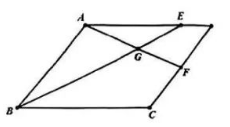$\text{A.}$ $\frac{3}{11} \vec{a}+\frac{4}{11} \vec{b}$ $\text{B.}$ $\frac{6}{11} \vec{a}+\frac{3}{11} \vec{b}$ $\text{C.}$ $\frac{4}{11} \vec{a}+\frac{5}{11} \vec{b}$ $\text{D.}$ $\frac{3}{11} \vec{a}+\frac{6}{11} \vec{b}$
【答案】 D

【解析】 易知 $\frac{E G}{A B}=\frac{3}{8}$, 由三点共线定理得 $\overrightarrow{A G}=\frac{3}{11} \overrightarrow{A B}+\frac{8}{11} \overrightarrow{A E}=\frac{3}{11} \overrightarrow{A B}+\frac{6}{11} \overrightarrow{A D}$ ，故选 D.